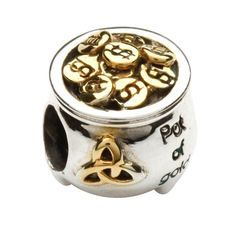# Treasure in gold and silver pot puzzle

Answered

There is a silver pot and a golden pot. One of these pots contains a treasure and the other one is empty.
Both of them have a label containing hints. Assume that you can determine from the labels which pot contains the treasure.

The labels on the pots are the following:
The silver pot: “This pot is empty.”
The golden pot: “Exactly one of these texts is true.”• 2 Answer(s)
Best answer

Answer – The Silver Pot contains the treasure

The statements:
(S) The silver pot: “This pot is empty.”
(G) The golden pot: “Exactly one of these texts is true.”

Premise: Let S be TRUE:

Assumption 1: ‘G’ is also true

This will contradict itself, because , as per the statement, only one of S / G can be true AND S is already true, which implies that G must be false, contradicting the assumption.

Assumption 2: G is False

Then either BOTH S and G should be TRUE OR both should be false. But since our premise is that S is true, this means G also must be true, again contradicting the assumption.

Thus, both the scenarios end up self-contradictory, which, in turn, implies that our premise must be wrong. So, ‘S’ must be false.

Then, G can be true, because only one statement (G) is true.

Hence the result: The label on silver pot is wrong => It is NOT  empty => contains the treasure.

• ### Your Answer

By posting your answer, you agree to the privacy policy and terms of service.